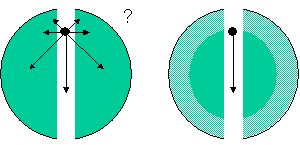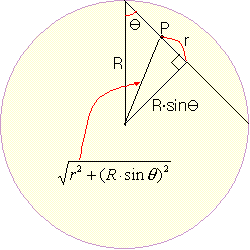# Gravity Train

Like this simulation, if you drill a hole in the earth and throw it in, you can send it to the other side of the earth.
This simulation ignores the effects of friction.
The earth is assumed to be a homogeneous solid sphere without rotation.
This simulation is just a thought experiment. Current technology can not make holes to pass through the earth.

## Newton’s Shell Theorem

What is the force of gravity acting on the particles shown below?Newton found that the sum of the gravity acting from the outer sphere is zero. Therefore, only the inner material of the sphere is involved in gravity.
Reorganizing Newton’s Shell Theorem looks like this:

The homogeneous shell exerts no gravity on the particles located inside it.

## Calculation of the force acting on an object in the earth tunnel

The force acting on the particles only affects the mass M ‘of the Earth inside the sphere with radius r.
The part of the outside of earth’s sphere exerts no force on the particles (Newton’s Shell Theorem).
Thus, the mass M ‘is:

$M’\, =\, \rho V\, =\, \rho \frac { 4 }{ 3 } { \pi r }^{ 3 }$

ρ is the density of the earth, assuming homogeneous.

According to Newton’s Shell Theorem, gravity acting on an object is inversely proportional to the square of the distance and proportional to the mass of the object.
The gravity F acting on the particle is:

$F\, =\, \frac { GmM’ }{ { r }^{ 2 } } \, =\, \frac { Gm\rho 4\pi { r }^{ 3 } }{ 3{ r }^{ 2 } } \, =\, (\frac { Gm\rho 4\pi }{ 3 } )r$

G is the gravity constant, m is the mass of the object.
The values ​​in parentheses are all fixed numbers that correspond to spring constants.
As a result, you can see that the force acting on the particles is completely proportional to the distance.

## Calculation of the period of oscillation

The period of the harmonic oscillation, such as an object suspended from a spring, is:

$T\, =\, 2\pi \sqrt { \frac { m }{ K } }$

K is a spring constant and you can substitute the value in () in the second equation. Therefore, the period of oscillation is calculated as follows:

\begin{align} T\, &=\, 2\pi \sqrt { \frac { m }{ K } } \, =\, 2\pi \sqrt { \frac { m }{ (\frac { Gm\rho 4\pi }{ 3 } ) } } \, =\, \sqrt { \frac { 3\pi }{ G\rho } } \\ &=\, \sqrt { \frac { 3\pi }{ 6.67\times { 10 }^{ -11 }{ m }^{ 3 }/kg\cdot { s }^{ 2 }\, \cdot \, 5.5\times { 10 }^{ 3 }kg/{ m }^{ 3 } } } \, \\ &\approx \, 5060\, s \end{align}

The round trip takes about 5060 seconds (= 84 minutes), so if this tunnel is used for postal delivery, the one-way delivery takes about 42 minutes. When the mail arrives, you need to catch it quickly. Otherwise, the mail will travel across the globe and return after 84 minutes.

## If you dug a tunnel with an angle,In the figure above, except for the shell, the mass M ‘inside P is:

$M’\, =\, \rho V\, =\, \rho \frac { 4\pi }{ 3 } \cdot { (\sqrt { { r }^{ 2 }+{ (R\cdot sin\theta ) }^{ 2 } } ) }^{ 3 }$

Thus, the force on P is:

\begin{align} F\, &=\, \frac { GmM’ }{ { (\sqrt { { r }^{ 2 }+{ (R\cdot sin\theta ) }^{ 2 } } ) }^{ 2 } } \cdot \frac { r }{ \sqrt { { r }^{ 2 }+{ (R\cdot sin\theta ) }^{ 2 } } } \\ &=\, \frac { Gm\rho \frac { 4\pi }{ 3 } \cdot { (\sqrt { { r }^{ 2 }+{ (R\cdot sin\theta ) }^{ 2 } } ) }^{ 3 } }{ { (\sqrt { { r }^{ 2 }+{ (R\cdot sin\theta ) }^{ 2 } } ) }^{ 2 } } \cdot \frac { r }{ \sqrt { { r }^{ 2 }+{ (R\cdot sin\theta ) }^{ 2 } } } \\ &=\, \frac { Gm\rho 4\pi }{ 3 } \cdot r \end{align}

Therefore, even if the tunnel is dug with an angle, the period does not change.